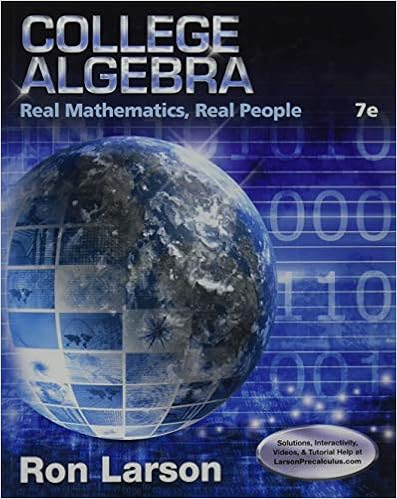MAT 334 practice problem - Math334 Practice Exercises 4 1...

• Homework Help
• 6

This preview shows page 1 - 3 out of 6 pages.

We have textbook solutions for you!
The document you are viewing contains questions related to this textbook.The document you are viewing contains questions related to this textbook.
Chapter 1 / Exercise 3
College Algebra: Real Mathematics, Real People
LarsonExpert Verified
Math334 - Practice Exercises 4 1 Jan 31 and Feb 2 1. By using sin z = e iz - e - iz 2 i , etc, show the followings (a) sin iz = i sinh z (b) sinh iz = i sin z (c) cos iz = cosh z (d) cosh iz = cos z 2. By using sin z = e iz - e - iz 2 i , etc, show the followings (a) sin( z + w ) = sin z cos w + sin w cos z . (b) cos( z + w ) = cos z cos w - sin z sin w . (c) sinh( z + w ) = sinh z cosh w + sinh w cosh z . (b) cosh( z + w ) = cosh z cosh w + sinh z sinh w . 3. By using sin z = e iz - e - iz 2 i , etc, show the followings (a) sin 2 z + cos 2 z = 1 (b) cosh 2 z - sinh 2 z = 1 (c) (sin z ) 0 = cos z (d) (cos z ) 0 = - sin z (e) (sinh z ) 0 = cosh z (f) (cosh z ) 0 = sinh z 4. Solve cosh x = 2 for x R . 5. Solve cosh z = 2 for z R . 6. Solve sin z = 1. 7. Solve e z = 4 + i . 8. Solve cos z = 1 + i . 9. Considering the notes for Feb 2, we tried to analyze how the function f ( z ) = sin z behaves. Let S = { a + yi } so that a is fixed and y is arbitrary. What is f ( S )? (Recall sin( x + iy ) = sin x cosh y + i cos x sinh y and cosh 2 y - sinh 2 y = 1) 1
We have textbook solutions for you!
The document you are viewing contains questions related to this textbook.The document you are viewing contains questions related to this textbook.
Chapter 1 / Exercise 3
College Algebra: Real Mathematics, Real People
LarsonExpert Verified
Math334 - Practice Exercises 5 1 Logarithms 1. Evaluate the followings (a) log 2 (b) Log (3 + 2 i ) (c) Log ( - 3 + 2) 2. While we know as multivalued functions, log z +log w = log( zw ), give an example so that Log z +Log w 6 = Log ( zw ). 3. Which of the following statements are true? Why? (a) e Log z = z (b) Log e z = z (c) e log z = z (d) log e z = z 4. Solve e 2 iz + 3 e iz + 2 = 0 for z . 5. What is the branch cut of Log ( z 2 + 3)? *6. We know that the branch cut of the principal branch of log z , i.e., Log z is the negative reals. Try to come up with an equation involving Log . (a) A branch of log z with the positive reals as branch cut, and takes value of at z = - 1. (Hint: Compute some examples of Log ( - z ) and compare to Log z .) (b) A branch of log z with positive imaginary axis as branch cut, and takes value of 0 at z = 1. (c) A branch of log z with negative imaginary axis as branch cut, and takes value of 0 at z = 1. 2 Complex Powers 1. Evaluate the followings (a) i 1 / 2 (b) i i
•••